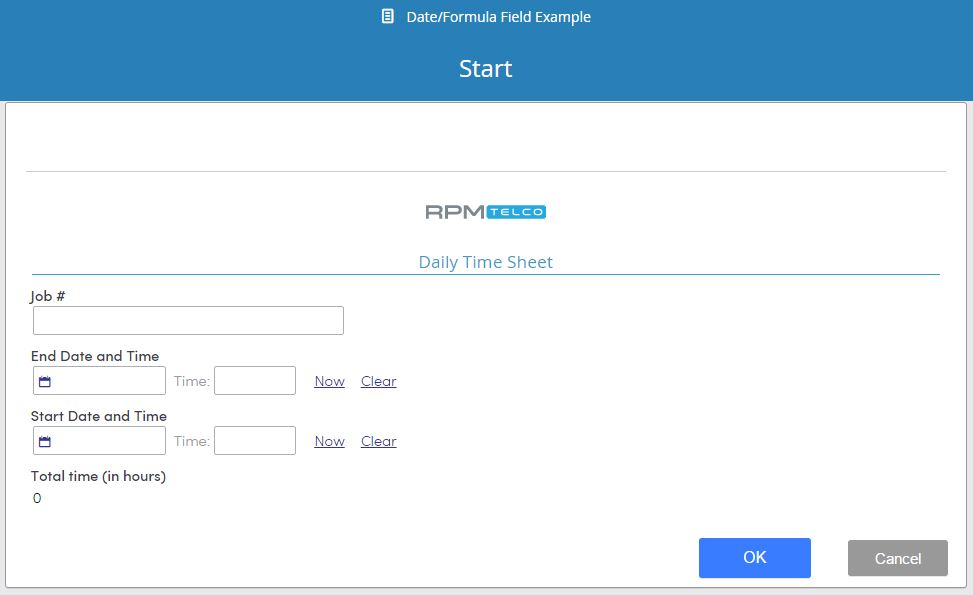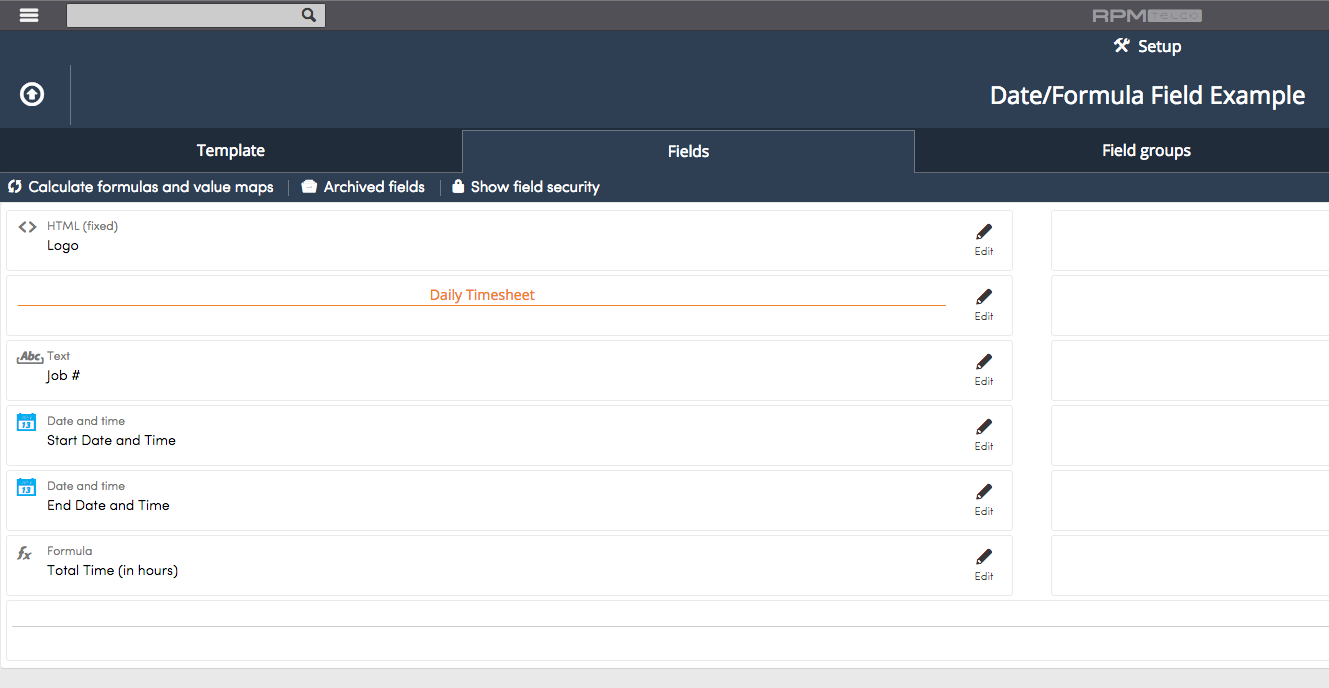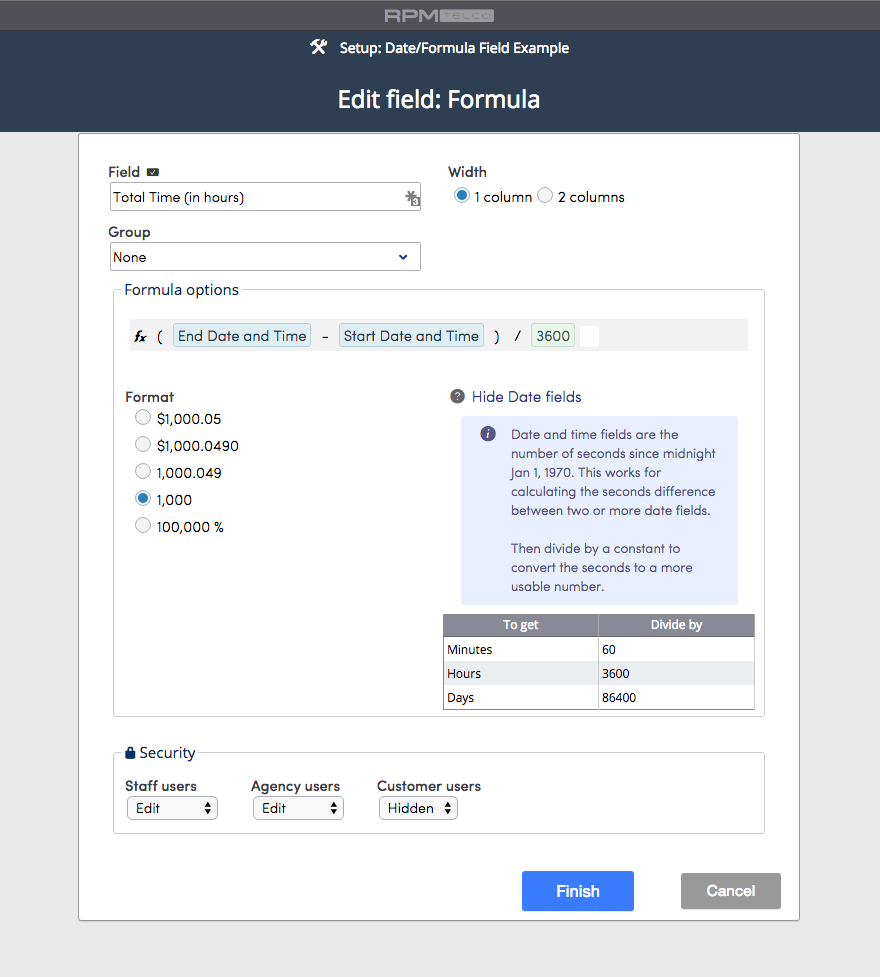Date and Date and Time fields can be used as inputs in Formula fields. When using a Date or Date and Time field in a formula the output is the number of seconds since midnight Jan 1, 1970. This means that when you use Date and Date and Time fields in a formula the output will be in seconds. Let's run through an example.

The screenshot below shows an example of a timesheet process. What's important in this process is keeping track of the Job # and the time an employee worked on that job for the day.The setup of this process is shown in the screenshot below.Of particular importance is the setup of the Formula field called Total Time (in hours). To calculate the hours worked, the field End Date and Time would be subtracted from the Start Date and Time field and then, the product divided by 3600 to get time in hours. See below.You can see in the screenshot above that we've included some help information and a handy conversion chart to use.